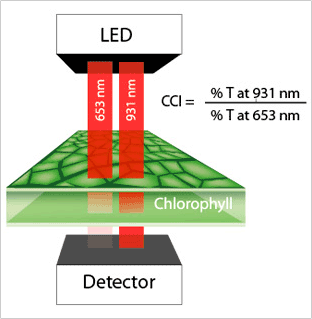# How the MC-100 Works### How the MC-100 Meter Works

Chlorophyll transmission is characteristically high in the near infrared range and very low in the red range because green plants absorb visible radiation for photosynthesis and transmit near infrared, which they do not use.

The MC-100 Chlorophyll Concentration Meter uses LEDs that emit specific wavelengths in the red and infrared ranges. The detector analyzes the ratio of the two wavelengths to determine chlorophyll concentration index (CCI). This index is then converted using equations derived by scientists at Utah State University (Parry et al., 2014) to output chlorophyll concentration in µmol per m2 of leaf surface.

For a brief video overview of how the MC-100 works, click here.

For an in depth explanation on the research and development of the MC-100, click here.

### Converting a µmol per m2 measurement to mg per m2

The leaves of higher plants include a mix of both chlorophyll a and b in about a 3 to 1 ratio (Parry et al., 2014). Chlorophyll a has a molar mass of 893.5 grams (g) per mole; chlorophyll b is slightly heavier at 907.5 grams per mole. The typical weighted average mass of both types of chlorophyll in plant leaves is very close to 900 grams per mole (this is equivalent to 900 micrograms per micromole; or 0.9 milligrams per micromole).

The reading(s) in µmoles per m2 can be converted to units of mg per m2 by multiplying by 0.9. For example, a reading of 400 µmoles per m2 is approximately 360 mg per m2.

### Converting from mg per m2 to µmol per m2

Using the average molar masses mention above, you can convert from mg per m2 to µmol per m2 by multiplying by 1.11 (1/0.9). For example, a reading of 350 mg per m2 is approximately 388.5 µmol per m2.